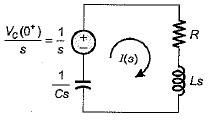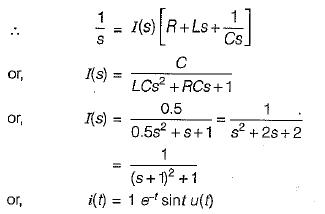Courses

# First Order RL & RC Circuits - 1

## 10 Questions MCQ Test Topicwise Question Bank for Electronics Engineering | First Order RL & RC Circuits - 1

Description
This mock test of First Order RL & RC Circuits - 1 for Electrical Engineering (EE) helps you for every Electrical Engineering (EE) entrance exam. This contains 10 Multiple Choice Questions for Electrical Engineering (EE) First Order RL & RC Circuits - 1 (mcq) to study with solutions a complete question bank. The solved questions answers in this First Order RL & RC Circuits - 1 quiz give you a good mix of easy questions and tough questions. Electrical Engineering (EE) students definitely take this First Order RL & RC Circuits - 1 exercise for a better result in the exam. You can find other First Order RL & RC Circuits - 1 extra questions, long questions & short questions for Electrical Engineering (EE) on EduRev as well by searching above.
QUESTION: 1

### The poles of the impedance Z(s) for the network shown in figure below will be real and coincident if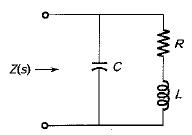Solution:

The impedance Z(s) of the given network is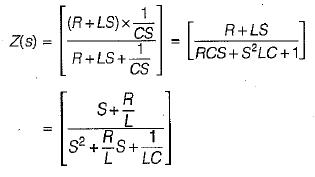The pofes are at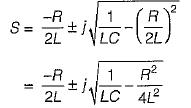Thus, the poles will be coincidence if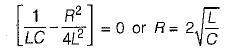QUESTION: 2

Solution:
QUESTION: 3

### A system is described by the transfer function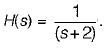The value of its step response at a very large time will be close to

Solution: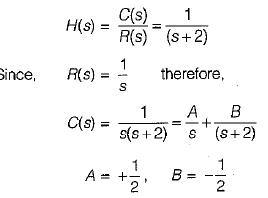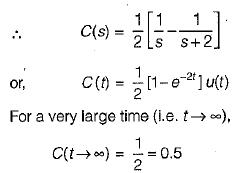QUESTION: 4

At a certain current, the energy stored in iron cored coil is 1000 J and its copper loss is 2000 W. The time constant (in second) of the coil is

Solution: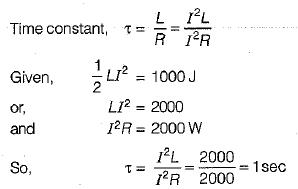QUESTION: 5

A ramp voltage vi(t) = 100t V is applied to a differentiator circuit with R = 5 kΩ and C=4μF.The maximum output voltage is

Solution:

Maximum output voltage of RC differentiator  circuit  =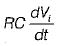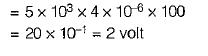QUESTION: 6

The Laplace transform of the waveform below is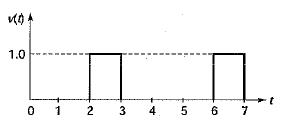Solution:

The given waveform can be expressed as

v(t) = u ( t - 2) - u ( t - 3) + u ( t - 6) - u ( t - 7)

Taking Laplace transform,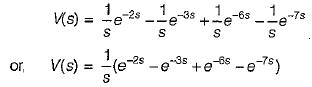QUESTION: 7

The initial and final values of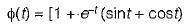are given by

Solution: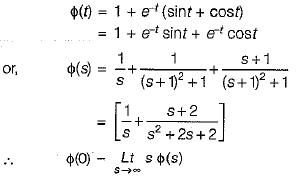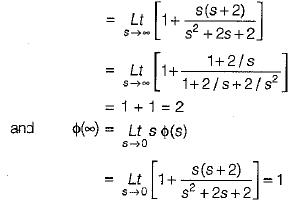QUESTION: 8

The Laplace transform of the ramp function shown below is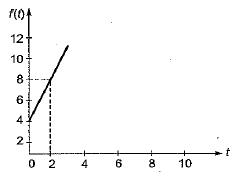Solution:

The equation of the given ramp function is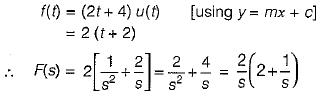QUESTION: 9

The poles and zeros of the transfer function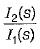for the circuit shown below are located at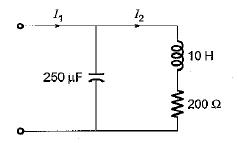Solution:

The given circuit can be redrawn as shown below.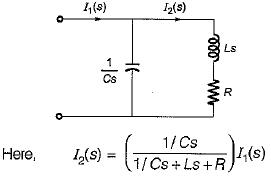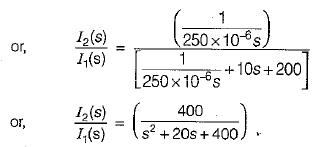Hence, poles are at: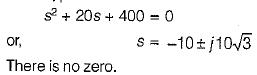QUESTION: 10

A capacitor of 0.5 F is initially charged to 1 volt and is subjected to discharge at t = 0 across a LR series circuit where 1 = 1 H; R = 2 Ω. The current i(t) for t> 0 is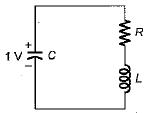Solution:

In Laplace domain, the given circuit can be drawn as shown below.